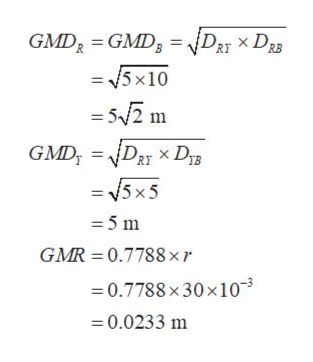# The three conductors of a 3-Φ transmission line are arranged in a horizantal plane and are 5m apart.The diameter of each conductor is 30mm.Determine the inductance per km of each conductor(line to neutral).Assume balanced load and R,Y,B phase sequence.Determine also the average inductance per phase for regularly transposed line.

Question
43 views

The three conductors of a 3-Φ transmission line are arranged in a horizantal plane and are 5m apart.The diameter of each conductor is 30mm.Determine the inductance per km of each conductor(line to neutral).Assume balanced load and R,Y,B phase sequence.Determine also the average inductance per phase for regularly transposed line.

check_circle

star
star
star
star
star
1 Rating
Step 1

Consider that the phase inductance of  phase conductor R,Y and B are LA, LB and LC respectively, geometrical mean distance of R, Y and B are GMDR, GMDY and  GMDB respectively. The geometrical mean radius of each conductor as GMR.

Step 2

Draw the diagram of  horizontal spacing of conductors. Consider that the radius of each conductor is r, and the distance between each conductor are DRY, DYB and DRB respectively as shown in figure.

Step 3

Calculate geometric mean distance and geometric mean radius of each conductor. Note that GMDR ...help_outlineImage TranscriptioncloseGMD2 GMD3 = NDRyX DzB = 5x10 = 5/2 m GMD D2 XD = 5x5 - 5 m GMR 0.7788xr 0.7788 x30x10 -0.0233 m fullscreen

### Want to see the full answer?

See Solution

#### Want to see this answer and more?

Solutions are written by subject experts who are available 24/7. Questions are typically answered within 1 hour.*

See Solution
*Response times may vary by subject and question.
Tagged in

### Electrical Engineering